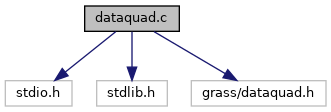GRASS GIS 7 Programmer's Manual  7.9.dev(2021)-e5379bbd7
`#include <stdio.h>`
`#include <stdlib.h>`
`#include <grass/dataquad.h>`Go to the source code of this file.

## Functions

struct triplequad_point_new (double x, double y, double z, double sm)

struct quaddataquad_data_new (double x_or, double y_or, double xmax, double ymax, int rows, int cols, int n_points, int kmax)

## Function Documentation

Add point to a given data.

Definition at line 143 of file dataquad.c.

 int quad_compare ( struct triple * point, struct quaddata * data )

Return the quadrant the point should be inserted in

Definition at line 98 of file dataquad.c.

 struct quaddata* quad_data_new ( double x_or, double y_or, double xmax, double ymax, int rows, int cols, int n_points, int kmax )

Initialize quaddata structure with given arguments

This is a constructor of the quaddata structure and it allocates memory. It also creates (and allocates memory for) the given number of points (given by kmax). The point attributes are set to zero.

Definition at line 62 of file dataquad.c.

Divide data into four new ones

Divides data into 4 new datas reinserting `data->points` in them by calling data function `quad_compare()` to determine were to insert. Returns array of 4 new datas (allocates memory).

Definition at line 237 of file dataquad.c.

Check if data needs to be divided

Checks if data needs to be divided. If `data->points` is empty, returns -1; if its not empty but there aren't enough points in data for division returns 0. Otherwise (if its not empty and there are too many points) returns 1.

Returns
1 if division is needed
0 if division is not needed
-1 if there are no points

Definition at line 219 of file dataquad.c.

Gets such points from data that lie within region determined by data_inter. Called by tree function `region_data()`.

Definition at line 323 of file dataquad.c.

Check intersection of two quaddata structures

Checks if region defined by data intersects the region defined by data_inter.

Definition at line 180 of file dataquad.c.